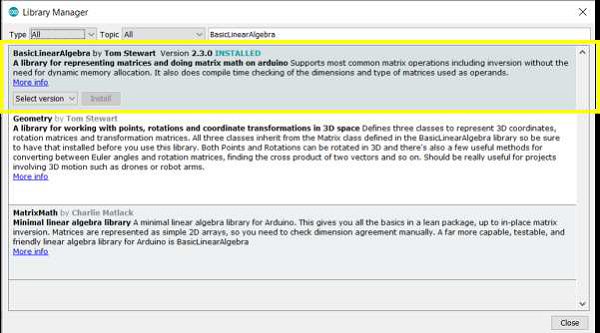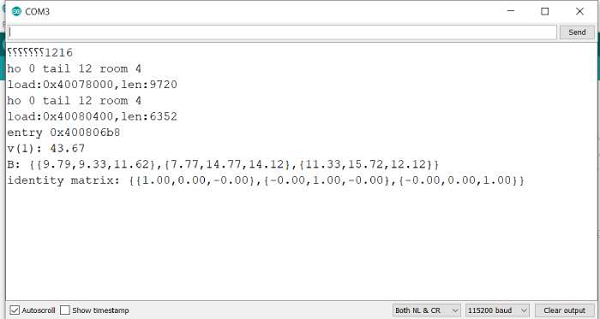# How to Perform Basic Linear Algebra on Arduino?

The BasicLinearAlgebra library helps represent matrices and perform matrix math on Arduino. To install it, search for 'BasicLinearAlgebra' in the Library Manager.Once installed, go to: File → Examples → BasicLinearAlgebra → HowToUse

As the name suggests, this example shows how to use this library. While the comments in this example do much of the explanation, here are a few pointers that help illustrate the use of this library −

You need to include the library and define the BLA namespace before getting started, as all the functions are wrapped up inside the BLA namespace.

#include <BasicLinearAlgebra.h>
using namespace BLA;

A matrix is defined using the following syntax −

BLA::Matrix<3,3> A;

A vector is defined using the following syntax:

BLA::Matrix<3> v;

To set every element of the matric/vector to a specific value, use the Fill() function.

v.Fill(0);

The row and column counts can be obtained using the .getRowCount() and .getColCount() respectively.

The inverse of a matrix is calculated using .Inverse() function.

To get the transpose, use the ~ operator. A_T = ~A.

A matrix can be initialized with the following syntax −

BLA::Matrix<3,3> B = {6.54, 3.66, 2.95,
3.22, 7.54, 5.12,
8.98, 9.99, 1.56};

Or using the Eigen-style comma notation −

A << 3.25, 5.67, 8.67,
4.55, 7.23, 9.00,
2.35, 5.73, 10.56;

You can horizontally concatenate two matrices using the || operator, and vertically using the && operator.

BLA::Matrix<3,6> AleftOfB = A || B;
BLA::Matrix<6,3> AonTopOfB = A && B;

There are several other operations (multiplication, addition, subtraction, etc.). You are encouraged to go through the entire example (it is heavily commented) to understand the syntax for these various operations. Also, you are strongly encouraged to go through the other examples that come along with this library. Several complex calculations are now possible onboard the Arduino, thanks to this library.

The purpose of this article was to make you aware that this library exists and what all is possible with this library. Also, needless to say, this library also allows printing of matrices on the Serial Monitor.

## Output

If you run this example, your Serial Monitor output looks like